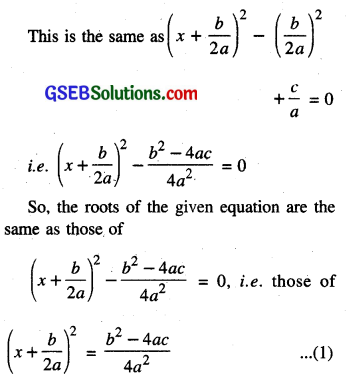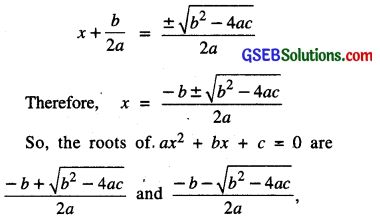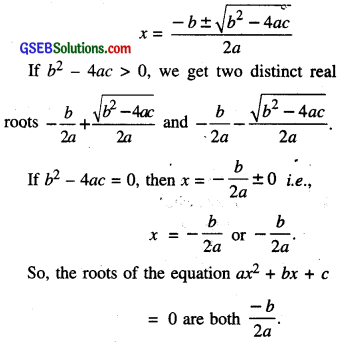# GSEB Class 10 Maths Notes Chapter 4 Quadratic Equations

This GSEB Class 10 Maths Notes Chapter 4 Quadratic Equations covers all the important topics and concepts as mentioned in the chapter.

## Quadratic Equations Class 10 GSEB Notes

We study about various forms of the Quadratic Polynomials. Let the general form of the quadratic polynomial be :
p (x) = ax2 + bx + c; a ≠ 0

Now, equate this polynomial to the zero, we get a Quadratic Equation i.e. p (x) = 0
⇒ ax2 + bx + c = 0 ; a ≠ 0

Quadratic Equations come up when we deal with many real life situations.
Many people believe that Babylonians were the first to solve quadratic equations. For instance, they knew how to find two positive numbers with a given positive sum and a given positive product, and this problem is equivalent to solving a quadratic equation of the form x2 – px + q – 0.

Greek mathematician Euclid developed geometrical approach for finding out lengths which, in our present-day terminology, are solutions of quadratic equations. Solving of quadratic equations, in general form, is often credited to ancient Indian mathematicians. In fact, Brahmagupta (A.D. 598-665) gave an explicit formula to solve a quadratic equation of the form ax2 + bx – c.

Later, Sridharacharya (A.D. 1025) derived a formula, now known as the quadratic formula, (as quoted by Bhaskara II) for solving quadratic equation by the method of completing the square. An Arab mathematician Al-Khwarizmi (about A.D. 800) also studied quadratic equations of different types. Abraham bar Hiyya Ha-Nasi, in his book ‘Liber embadorum’ published in Europe in A.D. 1145 gave complete solutions of different quadratic equations.

In this chapter, you will study quadratic equations, and various ways of finding their roots. You will also see some applications of quadratic equations in daily life situations.

Remark: When we write the terms of a Quadratic Equation in descending order of their degrees, is known as standard form of a Quadratic Equation i.e. ax2 + bx + c – 0; a ≠ 0Solution of a Quadratic Equation by Factorisation
In general. a real number α is called a root of the quadratic equation ax2 + bx + c = 0, a ≠ 0 aα2 + bα + c = 0. We also say that x = α is a solution of the quadratic equation, or that α-satisfies the quadratic equation. Note that the zeroes of the quadratic polynomial ax2 + bx + c and the roots of the quadratic equation ax2 + bx + c = 0 are the same.

We have observed, in Chapter 2, that a quadratic polynomial can have atmost two zeroes. So, any quadratic equation can have atmost two roots.

We have learnt in Class IX, how to factorise quadratic polynomials by splitting their middle terms. We shall use this knowledge for finding the roots of a quadratic equation.

Solution of a Quadratic Equation by completing the square Method to complete the squares

• Step 1. Take always constant term to R.H.S. of the given quadratic equation.
• Step 2. Divide both sides of equation by the coefficient of x2 i.e. make the coefficient of x2 unity.
• Step 3. Take coefficient of x and taking square of half the coefficient of x
[i.e. ($$\frac{1}{2}$$ coefficient of x)2]
• Step 4. Add the number obtained in step 3 to both sides of the given quadratic equation.
• Step 5. Now, solve the quadratic equation for given variable x.

ax2 + bx + c = 0 (a ≠ 0).
Dividing through out by a, we get: x2 + $$\frac{b}{a}$$x + $$\frac{c}{a}$$ =0If b2 – 4ac ≥ 0, then by taking the square roots in (1), we getif b2 – 4ac ≥ 0. In case if b2 – 4ac < 0, the equation will have no real mots.
Thus, If b2 – 4ac ≥ 0,then the roots of the quadratic equation ax2 + bx + c = 0 are given by $$\frac{-b \pm \sqrt{b^{2}-4 a c}}{2 a}$$
This formula for finding the roots of a quadratic equation is known as the quadratic formula

Nature of Roots
In the previous section, you have seen that the roots of the equation ax2 + bx + c = 0 are given byIf b2 – 4ac < 0, then there is no real number whose square is b2 – 4ac. Therefore, there are no real roots for the given quadratic equation in this case.
Therefore, we say that the quadratic equation ax2 + bx + c = 0 has two equal real roots in this case.

Since b2 – 4ac determines whether the quadratic equation ax2 + bx +c =0 has real roots or not, b2 – 4ac is called the discriminant of this quadratic equation.

So, a quadratic equation ax2 + bx + c = 0 has

• two distinct real roots if b2 – 4ac > 0,
• two equal real roots if b2 – 4ac = 0,
• no real roots if b2 – 4ac < 0.